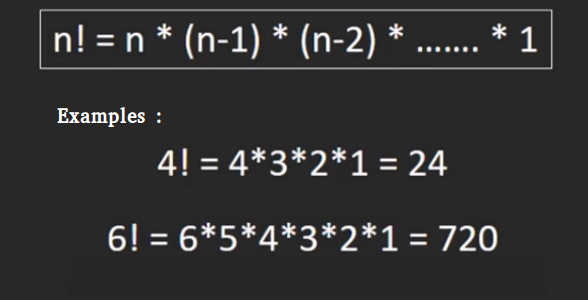Java Program for factorial of a number

• Difficulty Level : Medium
• Last Updated : 20 Mar, 2018

Factorial of a non-negative integer, is multiplication of all integers smaller than or equal to n. For example factorial of 6 is 6*5*4*3*2*1 which is 720.Recursive :

 // Java program to find factorial of given numberclass Test{    // method to find factorial of given number    static int factorial(int n)    {        if (n == 0)          return 1;                  return n*factorial(n-1);    }          // Driver method    public static void main(String[] args)     {        int num = 5;        System.out.println("Factorial of "+ num + " is " + factorial(5));    }}

Iterative Solution:

 // Java program to find factorial of given numberclass Test{    // Method to find factorial of given number    static int factorial(int n)    {        int res = 1, i;        for (i=2; i<=n; i++)            res *= i;        return res;    }          // Driver method    public static void main(String[] args)     {        int num = 5;        System.out.println("Factorial of "+ num + " is " + factorial(5));    }}

One line Solution (Using Ternary operator):

 // Java program to find factorial// of given numberclass Factorial {    int factorial(int n)  {      // single line to find factorial    return (n == 1 || n == 0) ? 1 : n * factorial(n - 1);    }      // Driver Code  public static void main(String args[])       {    Factorial obj = new Factorial();    int num = 5;    System.out.println("Factorial of " + num +                       " is " + obj.factorial(num));   }}// This code is contributed by Anshika Goyal.

The above solutions cause overflow for small numbers. Please refer factorial of large number for a solution that works for large numbers.

Please refer complete article on Program for factorial of a number for more details!

My Personal Notes arrow_drop_up